在日常工作中，常常会用到需要周期性执行的任务，一种方式是采用 Linux 系统自带的 crond 结合命令行实现。另外一种方式是直接使用Python。

当每隔一段时间就要执行一段程序，或者往复循环执行某一个任务，这就需要使用定时任务来执行程序。比如在实现对某个目标进行爬虫的话，需要用到实时任务。

python中常用的定时任务主要有以下8中方法：

1. while True：+sleep()
3. Timeloop库执行定时任务
4. 调度模块sched
5. 调度模块schedule
6. 任务框架APScheduler
7. 分布式消息系统celery执行定时任务
8. 使用windows自带的定时任务

``````from datetime import datetime

now = datetime.now()
ts = now.strftime("%Y-%m-%d %H:%M:%S")
print(ts)
``````

## 1、利用while True：+sleep()实现定时任务

最简单的方式应该就是使用time模块来实现定时任务，在循环里面放入要执行的任务，然后sleep一段时间再执行。实现令当前执行的线程暂停 n秒后再继续执行。所谓暂停，即令当前线程进入阻塞状态，当达到 sleep() 函数规定的时间后，再由阻塞状态转为就绪状态，等待 CPU 调度。

``````from datetime import datetime
import time

now = datetime.now()
ts = now.strftime("%Y-%m-%d %H:%M:%S")
print(ts)

def func():
while True:
time.sleep(3)

func()
``````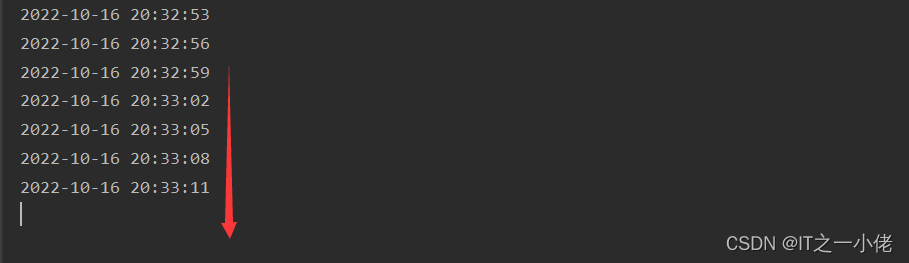timer最基本理解就是定时器，可以启动多个定时任务，这些定时器任务是异步执行，所以不存在等待顺序执行问题。

Timer方法说明
Timer(interval, function, args=None, kwargs=None)创建定时器
cancel()取消定时器
start()使用线程方式执行
join(self, timeout=None)等待线程执行结束

``````from datetime import datetime

now = datetime.now()
ts = now.strftime("%Y-%m-%d %H:%M:%S")
print(ts)

def func():
t = Timer(3, func)
t.start()

func()
``````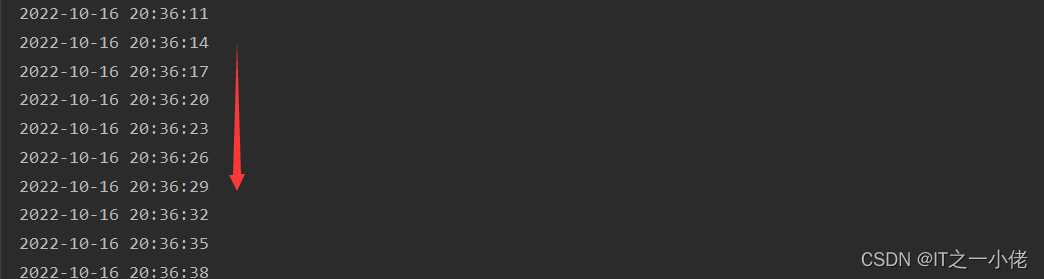``sys.setrecursionlimit(100000000)``

## 3、使用Timeloop库执行定时任务

Timeloop是一个库，可用于运行多周期任务。这是一个简单的库，使用decorator模式在线程中运行标记函数。

``````from datetime import datetime, timedelta
from timeloop import Timeloop

tl = Timeloop()

now = datetime.now()
ts = now.strftime("%Y-%m-%d %H:%M:%S")
print(ts + '333!')

now = datetime.now()
ts = now.strftime("%Y-%m-%d %H:%M:%S")
print(ts + "555555!")

@tl.job(interval=timedelta(seconds=2))
def sample_job_every_2s():

@tl.job(interval=timedelta(seconds=5))
def sample_job_every_5s():
``````

## 4、利用调度模块sched实现定时任务

sched是一种调度（延时处理机制）。sched模块实现了一个通用事件调度器，在调度器类使用一个延迟函数等待特定的时间，执行任务。同时支持多线程应用程序，在每个任务执行后会立刻调用延时函数，以确保其他线程也能执行。

scheduler对象主要方法：

• enter(delay, priority, action, argument)，安排一个事件来延迟delay个时间单位。
• cancel(event)：从队列中删除事件。如果事件不是当前队列中的事件，则该方法将跑出一个ValueError。
• run()：运行所有预定的事件。这个函数将等待(使用传递给构造函数的delayfunc()函数)，然后执行事件，直到不再有预定的事件。

``````import sched
import time
from datetime import datetime

# 初始化sched模块的scheduler类
# 第一个参数是一个可以返回时间戳的函数，第二个参数可以在定时未到达之前阻塞。
schedule = sched.scheduler(time.time, time.sleep)

now = datetime.now()
ts = now.strftime("%Y-%m-%d %H:%M:%S")
print(ts)

def func(inc=3):
# enter四个参数分别为：
# 间隔事件、优先级（用于同时间到达的两个事件同时执行时定序）、被调用触发的函数、给该触发函数的参数（tuple形式）
schedule.run()

func()
``````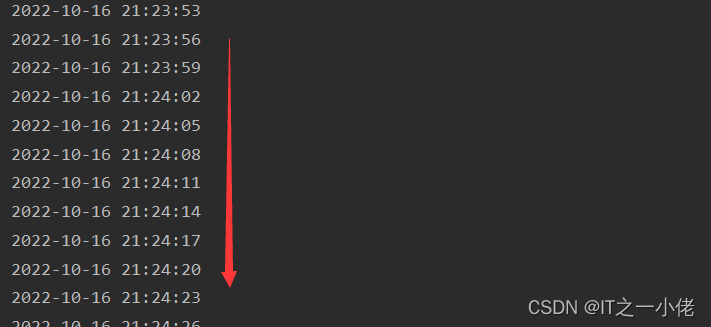## 5、利用调度模块schedule实现定时任务

schedule是一个第三方轻量级的任务调度模块，可以按照秒，分，小时，日期或者自定义事件执行时间。

``````import schedule
from datetime import datetime

now = datetime.now()
ts = now.strftime("%Y-%m-%d %H:%M:%S")
print(ts)

now = datetime.now()
ts = now.strftime("%Y-%m-%d %H:%M:%S")
print(ts + '666!')

def func():
# 清空任务
schedule.clear()
# 创建一个按3秒间隔执行任务
# 创建一个按2秒间隔执行任务
while True:
schedule.run_pending()

func()
``````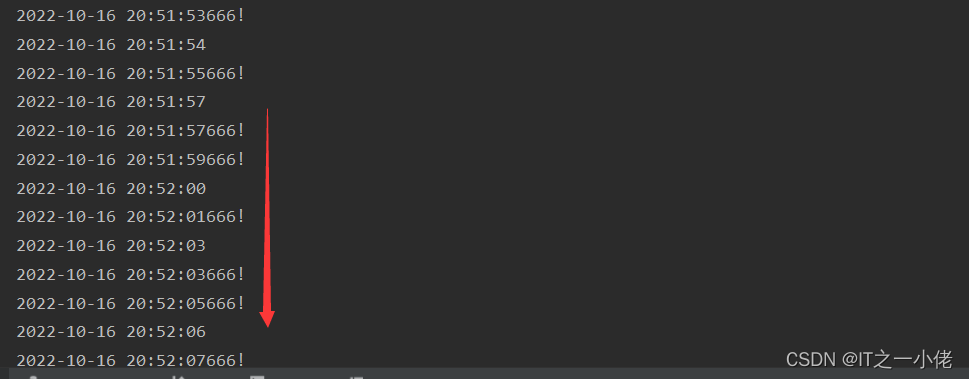## 6、利用任务框架ASPcheduler实现定时任务

APScheduler是Python的一个定时任务框架，用于执行周期或者定时任务，该框架不仅可以添加、删除定时任务，还可以将任务存储到数据库中，实现任务的持久化，使用起来非常方便。

``````from datetime import datetime
from apscheduler.schedulers.blocking import BlockingScheduler

now = datetime.now()
ts = now.strftime("%Y-%m-%d %H:%M:%S")
print(ts)

now = datetime.now()
ts = now.strftime("%Y-%m-%d %H:%M:%S")
print(ts + '666!')

def func():
# 创建调度器BlockingScheduler()
scheduler = BlockingScheduler()
# 添加任务，时间间隔为5秒
scheduler.start()

func()
``````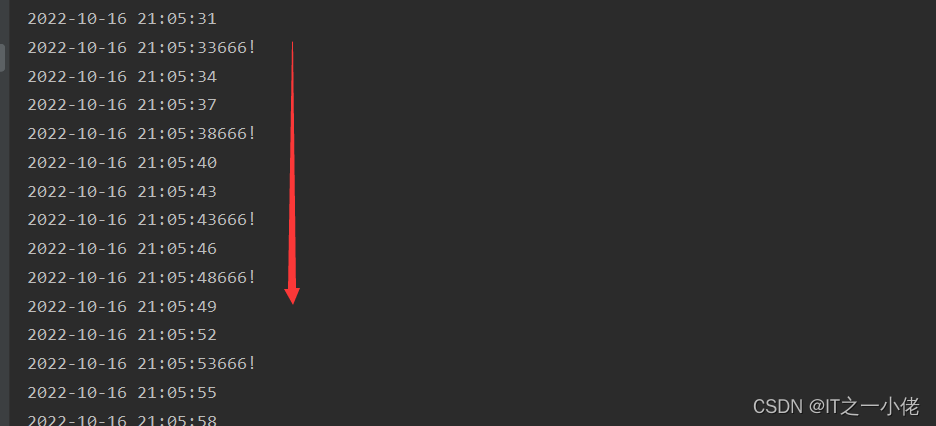## 7、使用分布式消息系统celery执行定时任务

Celery是一个简单，灵活，可靠的分布式系统，用于处理大量消息，同时为操作提供维护此类系统所需的工具, 也可用于任务调度。Celery 的配置比较麻烦，如果你只是需要一个轻量级的调度工具，Celery 不会是一个好选择。

Celery 是一个强大的分布式任务队列，它可以让任务的执行完全脱离主程序，甚至可以被分配到其他主机上运行。我们通常使用它来实现异步任务（async task）和定时任务（crontab）。 异步任务比如是发送邮件、或者文件上传, 图像处理等等一些比较耗时的操作 ，定时任务是需要在特定时间执行的任务。

## 8、使用windows自带的定时任务

略。这儿不做细述！